# RD Sharma Solutions for Class 6 Maths Chapter 2: Playing with Numbers Exercise 2.4

## RD Sharma Solutions for Class 6 Maths Exercise 2.4 PDF

Mathematics is one of the challenging subjects and scoring well mainly requires a lot of practice. Students can now practice the RD Sharma Solutions for Class 6 which are provided here. These questions are solved based on the current CBSE syllabus and guidelines. The primary aim of creating chapter wise and exercise wise Solutions is to make the students familiar with the concepts. Each solution is provided with better illustrations in order to make students understand the concepts in a shorter duration. RD Sharma Solutions for Class 6 Maths Chapter 2 Playing with Numbers Exercise 2.4 are provided here.

## RD Sharma Solutions for Class 6 Maths Chapter 2: Playing with Numbers Exercise 2.4 Download PDF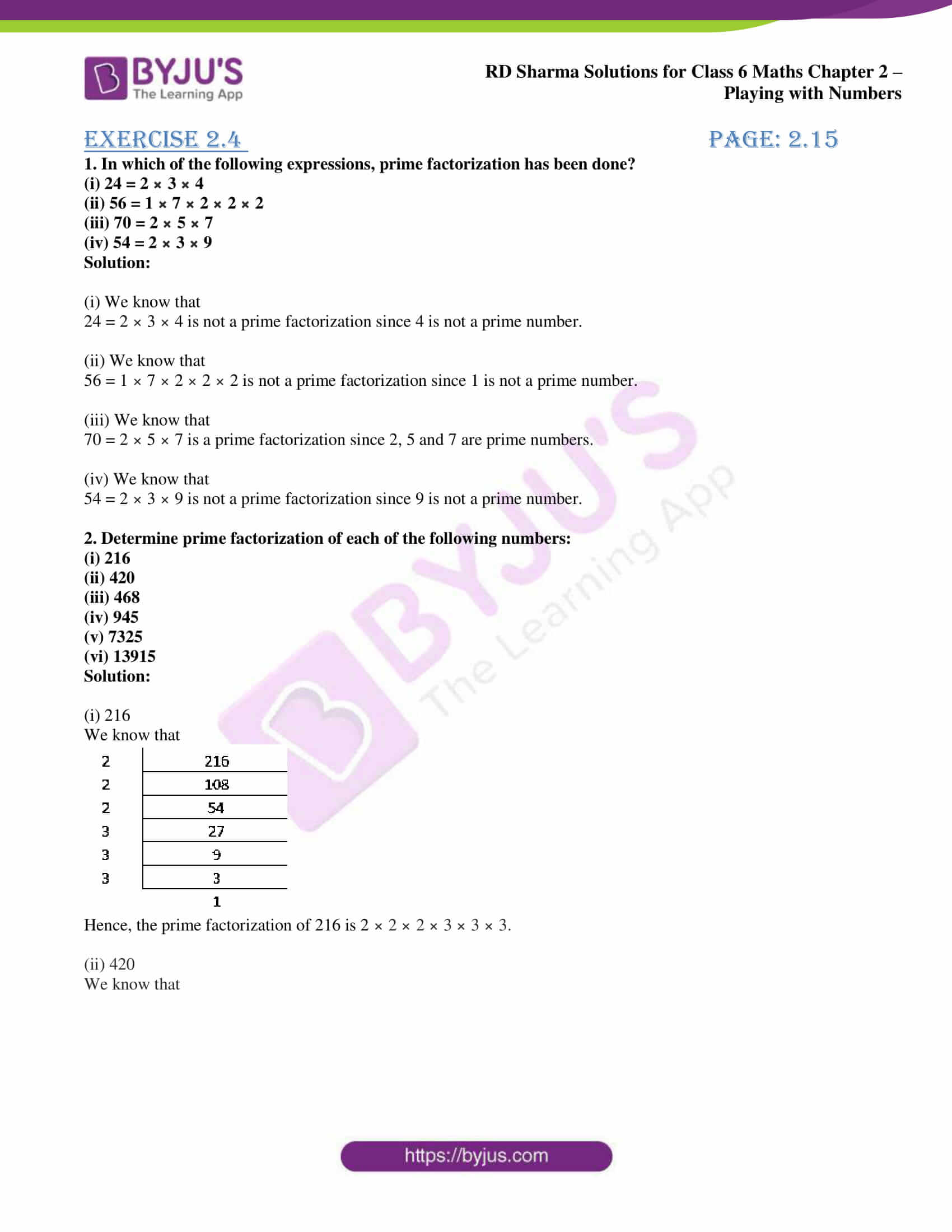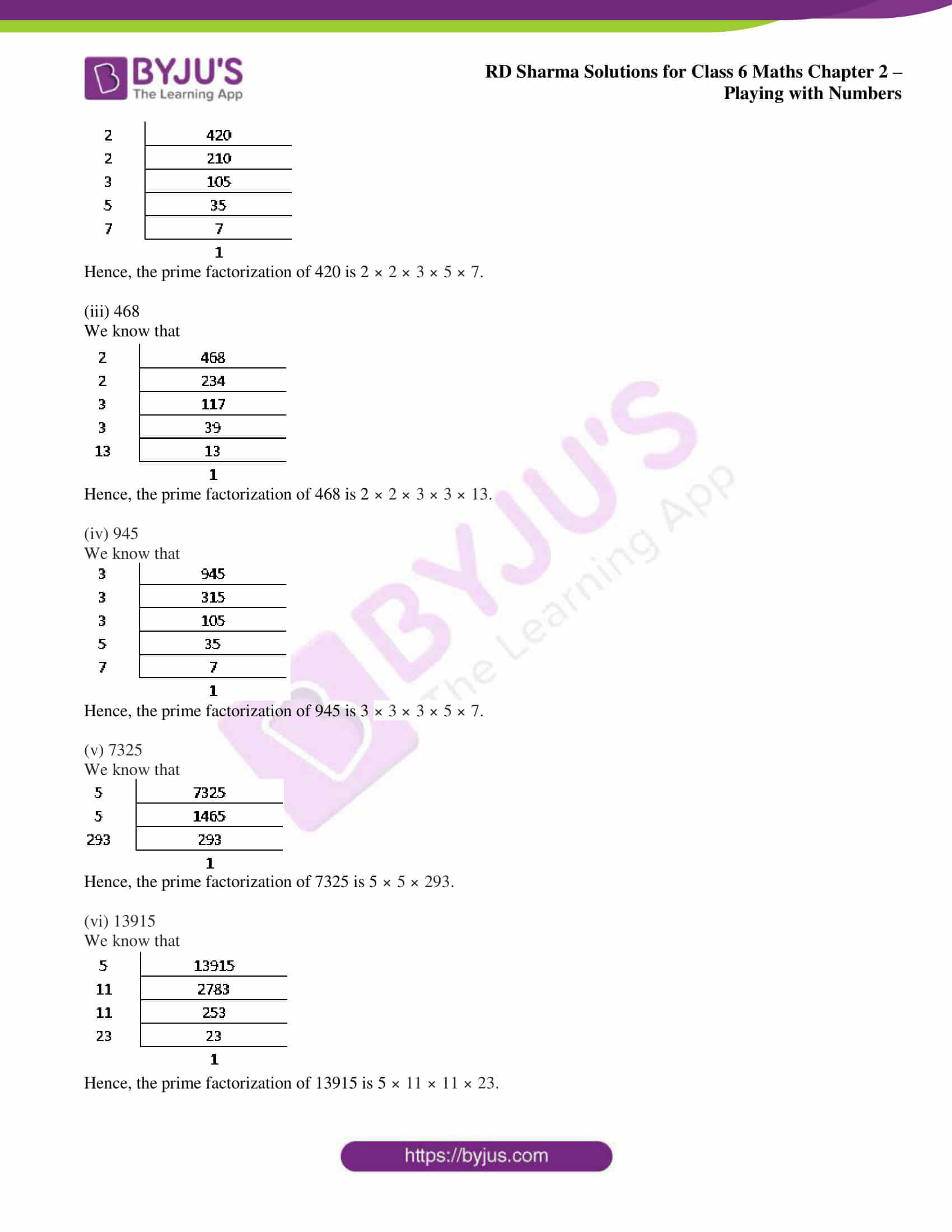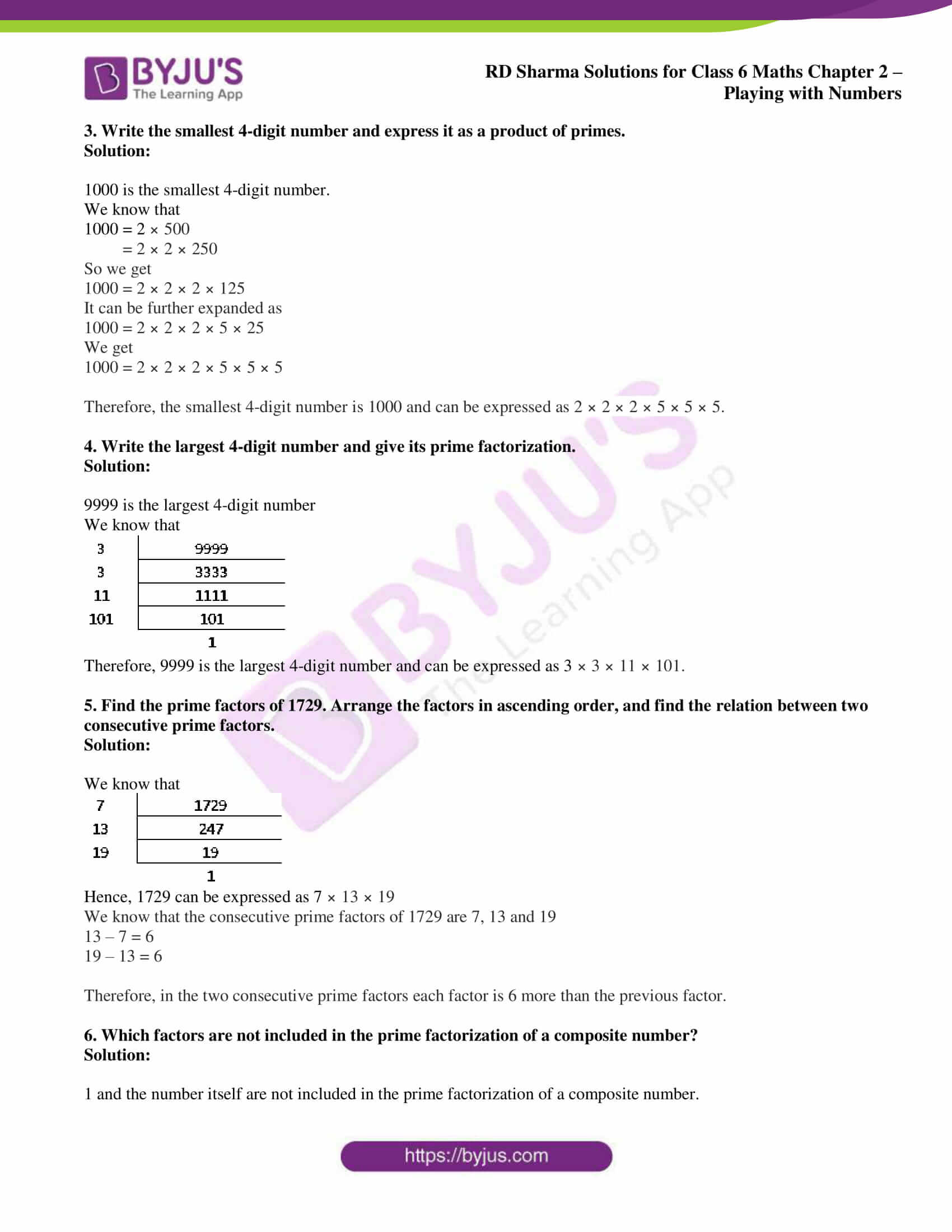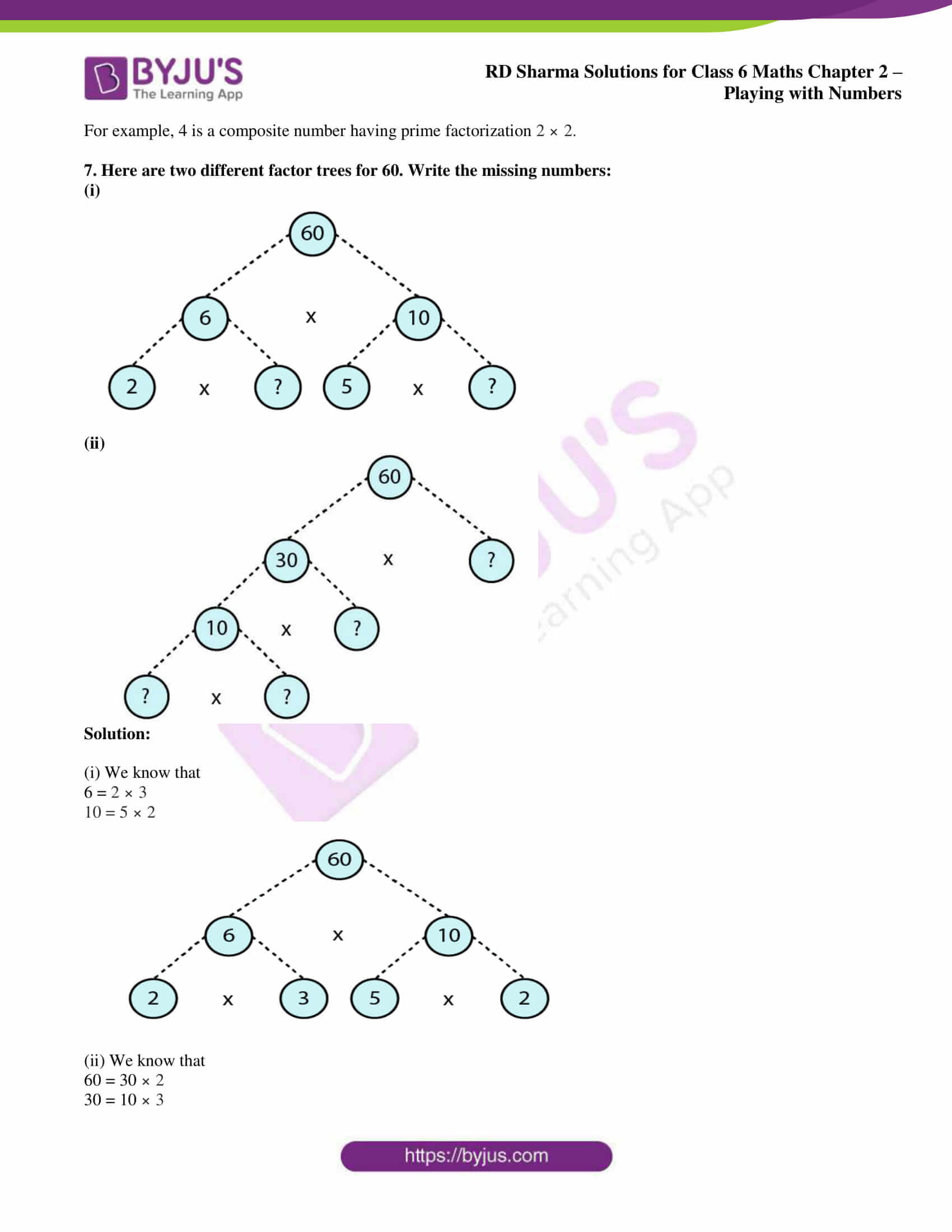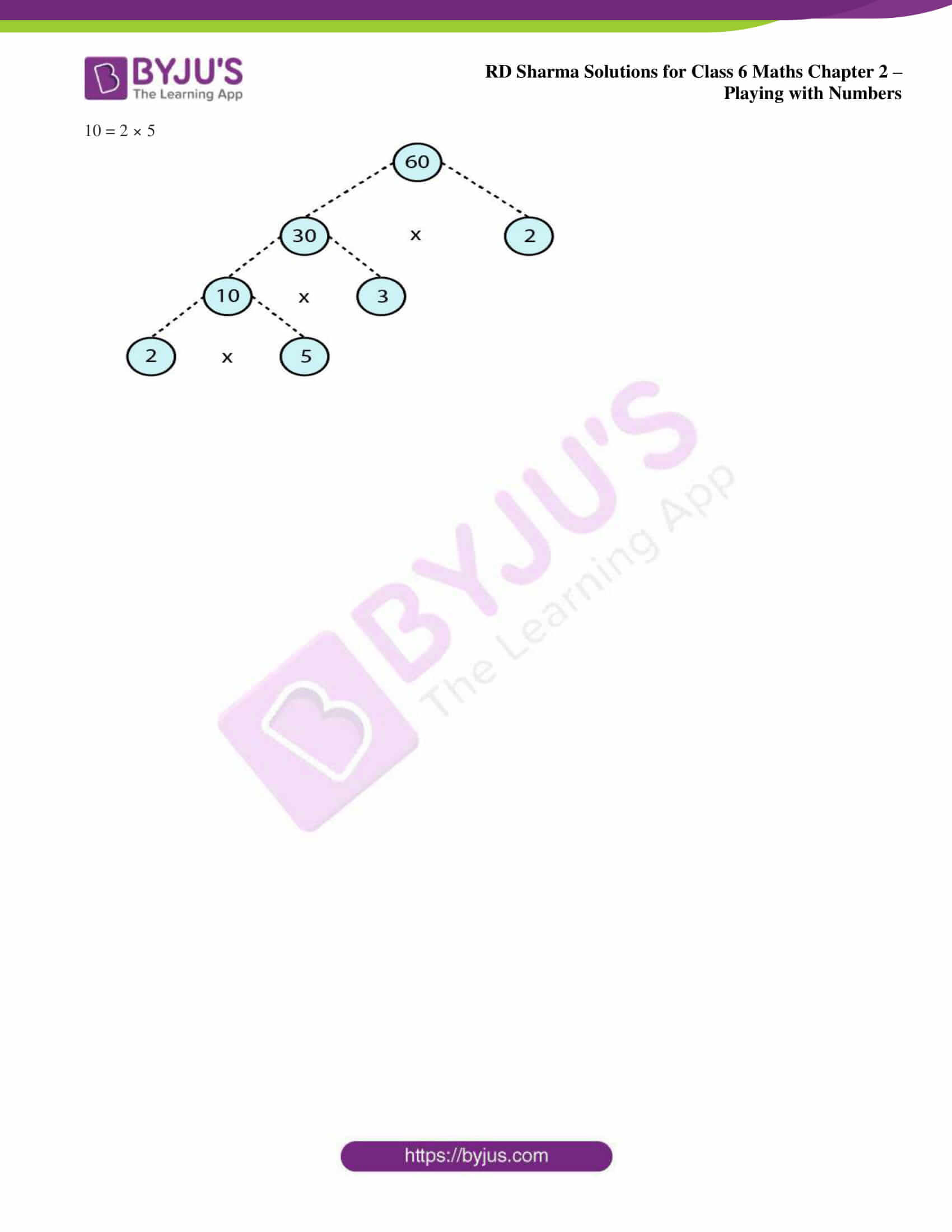## Access answers to Maths RD Sharma Solutions for Class 6 Chapter 2: Playing with Numbers Exercise 2.4

1. In which of the following expressions, prime factorization has been done?

(i) 24 = 2 × 3 × 4

(ii) 56 = 1 × 7 × 2 × 2 × 2

(iii) 70 = 2 × 5 × 7

(iv) 54 = 2 × 3 × 9

Solution:

(i) We know that

24 = 2 × 3 × 4 is not a prime factorization since 4 is not a prime number.

(ii) We know that

56 = 1 × 7 × 2 × 2 × 2 is not a prime factorization since 1 is not a prime number.

(iii) We know that

70 = 2 × 5 × 7 is a prime factorization since 2, 5 and 7 are prime numbers.

(iv) We know that

54 = 2 × 3 × 9 is not a prime factorization since 9 is not a prime number.

2. Determine prime factorization of each of the following numbers:

(i) 216

(ii) 420

(iii) 468

(iv) 945

(v) 7325

(vi) 13915

Solution:

(i) 216

We know that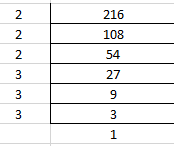Hence, the prime factorization of 216 is 2 × 2 × 2 × 3 × 3 × 3.

(ii) 420

We know that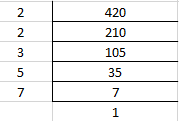Hence, the prime factorization of 420 is 2 × 2 × 3 × 5 × 7.

(iii) 468

We know that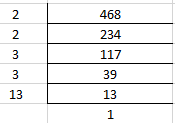Hence, the prime factorization of 468 is 2 × 2 × 3 × 3 × 13.

(iv) 945

We know that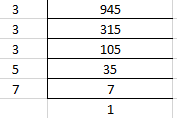Hence, the prime factorization of 945 is 3 × 3 × 3 × 5 × 7.

(v) 7325

We know that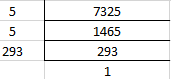Hence, the prime factorization of 7325 is 5 × 5 × 293.

(vi) 13915

We know that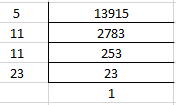Hence, the prime factorization of 13915 is 5 × 11 × 11 × 23.

3. Write the smallest 4-digit number and express it as a product of primes.

Solution:

1000 is the smallest 4-digit number.

We know that

1000 = 2 × 500

= 2 × 2 × 250

So we get

1000 = 2 × 2 × 2 × 125

It can be further expanded as

1000 = 2 × 2 × 2 × 5 × 25

We get

1000 = 2 × 2 × 2 × 5 × 5 × 5

Therefore, the smallest 4-digit number is 1000 and can be expressed as 2 × 2 × 2 × 5 × 5 × 5.

4. Write the largest 4-digit number and give its prime factorization.

Solution:

9999 is the largest 4-digit number

We know that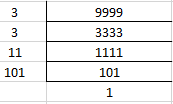Therefore, 9999 is the largest 4-digit number and can be expressed as 3 × 3 × 11 × 101.

5. Find the prime factors of 1729. Arrange the factors in ascending order, and find the relation between two consecutive prime factors.

Solution:

We know that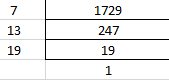Hence, 1729 can be expressed as 7 × 13 × 19

We know that the consecutive prime factors of 1729 are 7, 13 and 19

13 – 7 = 6

19 – 13 = 6

Therefore, in the two consecutive prime factors each factor is 6 more than the previous factor.

6. Which factors are not included in the prime factorization of a composite number?

Solution:

1 and the number itself are not included in the prime factorization of a composite number.

For example, 4 is a composite number having prime factorization 2 × 2.

7. Here are two different factor trees for 60. Write the missing numbers:

(i)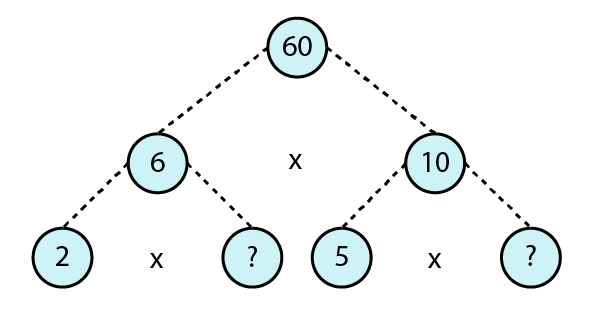(ii)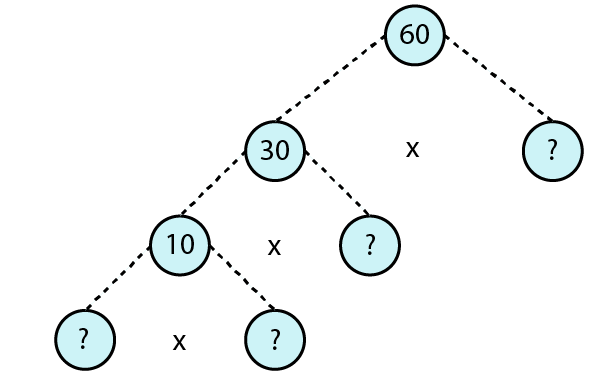Solution:

(i) We know that

6 = 2 × 3

10 = 5 × 2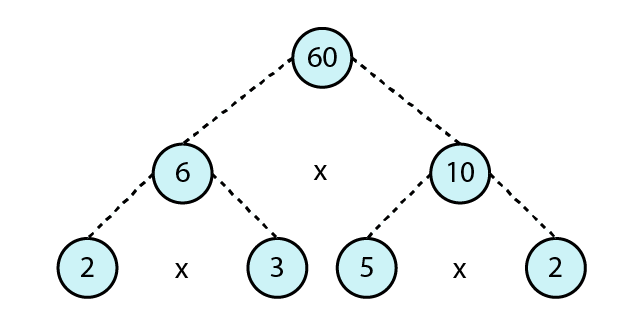(ii) We know that

60 = 30 × 2

30 = 10 × 3

10 = 2 × 5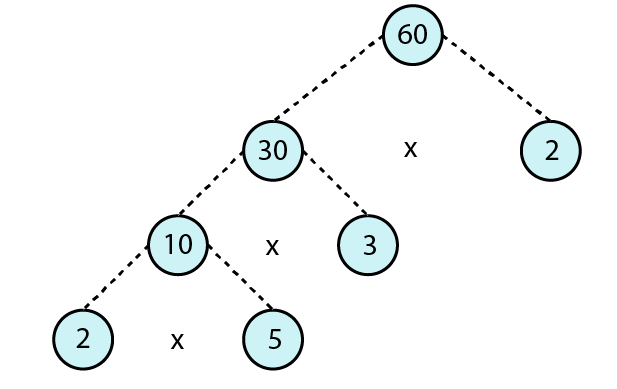### RD Sharma Solutions for Class 6 Maths Chapter 2 – Playing with Numbers Exercise 2.4

RD Sharma Solutions Class 6 Maths Chapter 2 Playing with Numbers Exercise 2.4 consists of answers for the fourth exercise along with illustrative examples, which help in understanding the concept of prime factorization.

### Key features of RD Sharma Solutions for Class 6 Maths Chapter 2: Playing with Numbers Exercise 2.4

• The solutions created by subject experts help students to practice well and revise for the exams.
• By solving ample number of problems in each exercise, students can self assess their conceptual knowledge and areas of weaknesses.
• RD Sharma Solutions with explanations mainly help students in solving difficult problems more efficiently.
• The students will understand the type of questions which might appear in the exam and the methods which can be used to solve them.